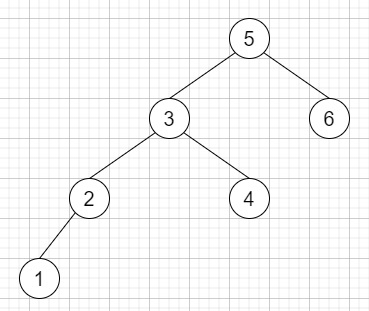# Inorder Successor in BST II in C++

Suppose we have a node in a binary search tree, we have to find the in-order successor of that node in the BST. If there is no in-order successor, return null. As we know that the successor of a node is the node with the smallest key greater than value of node.

We will have direct access to the node but not to the root of the tree. Here each node will have a reference to its parent node. Below is the definition for Node −

class Node {
public int val;
public Node left;
public Node right;
public Node parent;
}

If the input is like −and node is 2, then the output will be 3.

To solve this, we will follow these steps −

• if right of node is not null, then −

• node := right of node

• while left of node is not null, do −

• node := left of node

• return node

• while (parent of node is not null and node is not equal to left of parent of node), do −

• node := parent of node

• parent of return node

## Example

Let us see the following implementation to get a better understanding −

Live Demo

#include <bits/stdc++.h>
using namespace std;
class Node {
public:
int val;
Node* left;
Node* right;
Node* parent;
Node(int v, Node* par = NULL){
val = v;
left = NULL;
right = NULL;
parent = par;
}
};
class Solution {
public:
Node* inorderSuccessor(Node* node) {
if (node->right) {
node = node->right;
while (node->left)
node = node->left;
return node;
}
while (node->parent && node != node->parent->left) {
node = node->parent;
}
return node->parent;
}
};
main(){
Solution ob;
Node *root = new Node(5);
root->left = new Node(3, root);
root->right = new Node(6, root);
root->left->left = new Node(2, root->left);
root->left->right = new Node(4, root->left);
root->left->left->left = new Node(1, root->left->left);
cout << (ob.inorderSuccessor(root->left->left))->val;
}

## Input

Node *root = new Node(5);
root->left = new Node(3, root);
root->right = new Node(6, root);
root->left->left = new Node(2, root->left);
root->left->right = new Node(4, root->left);
root->left->left->left = new Node(1, root->left->left);
(ob.inorderSuccessor(root->left->left))->val

## Output

3